Bending Analysis of Symmetrically Laminated Plates

1 Department of Civil Engineering, University of Bechar, Bechar 08000, Algeria.

2 Department of Mechanical Engineerin , University of Bechar, Bechar 08000, Algeria.

E-mail: bouazza_mokhtar@yahoo.fr

Abstract

In the classical plate theory, it is assumed that the plane cross sections initially normal to the plate midsurface before deformation remain plane and normal to that surface during deformation. This is the result of neglecting the transverse shear strains. However, in thick and moderately thick laminated plates, significant transverse shear strains occur, and the theory gives inaccurate results for the plates. So, it is obvious that the shear strains have to be taken into account. There are numerous theories of plates and laminated plates that include the transverse shear strains. One of them is the Reissner and Midlin theory , known as the first-order shear deformation theory, which defines the displacement field as linear variations of midplane displacements. This theory, where the relation between the resultant shear forces and the shear strains is obtained by using shear correction factors, has some advantages due to its simplicity and low computational cost. Some other plate theories, namely the higher-order shear deformation theories, include the effect of transverse shear strains . For example, the theory developed by Reddy allows not only for the transverse shear strains, but also for parabolic variations in the strains across the plate thickness, and thus there is no need to use shear correction coefficients in computing the shear stresses. The present stud is a survey of plate bending of cross-ply laminate by using the finite element method (F.E.M). Using ANSYS, the most known software in the domain for it, two types of modeling are proposed: the first is modeling using a type of shell element, Shell 99 and the second is an approach based on a of type solid element, Solid 46. The results obtained are compared with the results of the theory of Reddy.

Keywords

Laminate; Composite; Plate; Third-Order Shear Deformation Plate Theory; Finite Element Method.

Introduction

Research on thin plate theory is abundant. It has been based on the Kirchhoff's classical plate theory (CPT)  assuming that normals to the mid-plane before deformation remain straight and normal to the plane after deformation so the effects of the transverse shear strain are neglected. Such assumption restricts the model to thin plates or plates whose elastic modulus to shear modulus ratios is not very large. However, increasingly many engineering applications, such as cylinders for nuclear reactors, require the use of thick-walled structures for which the Kirchhoff's CPT is inadequate because it underestimates deflections and overestimates vibration frequencies and buckling loads. A thorough understanding of the dynamics of thick-walled structures is therefore conducive to an efficient design.

The study of thick plates can be traced back to mid 1940s and early 1950s pioneered by Reissner [2, 3] and Mindlin . The Reissner-Mindlin’s first-order shear deformation plate theory (FSDT) assumes first-order displacement functions with a shear correction factor for alleviating the discrepancy of having non-zero transverse shear strain on the top and bottom surfaces. This theory was employed by Whitney and Pagano to study vibration and bending of anisotropic plates  and by Liew et al. to analyze a thick plate with a maximum of 20% of thickness-to-width ratio . Various higher-order theories were proposed which include the second-order shear deformation formulation of Whitney and Sun [7,8], Gautham and Ganesan , and the third-order shear deformation theory (TSDT) [10-18]. One of the earliest applications of TSDT is by Ambartsumyan  who formulated shear stresses txz and tyz as parabolic distributions through the thickness. In addition, he also considered non-zero txz and tyz on the top and bottom surfaces so that the presence of shear loading is possible. Of the many higher order plate theories, the TSDT of Levinson  and Reddy and his associates [14,20] is, perhaps, the most widely adopted model in the study of laminated plates. It has received intensive interest in the analysis owing to the elimination of a shear correction factor. It is variationally consistent among the third order theories. It, however, imposes a restriction that transverse shear stress vanishes on the top and bottom surfaces of the plate. Thus, the displacement cubic variations through the thickness are constrained by the transverse displacement and the linear rotations. Effective general force of higher orders must be introduced to achieve the variational consistency along the boundaries. Such requirement, although reasonable in many engineering applications, is not valid when the plate is subject to shear traction parallel to the surface.

Shimpiand Patel  presented a two variable refined plate theory (RPT) for orthotropic plates. This theory which looks like higher-order theory uses only two unknown functions in order to derive two governing equations for orthotropic plates. The most interesting feature of this theory is that it does not require shear correction factor, and has strong similarities with the CPT in some aspects such as governing equation, boundary conditions and moment expressions. The accuracy of this theory has been demonstrated for static bending and free vibration behaviours of plates by Shimpi and Patel , therefore, it seems to be important to extend this theory to the static buckling behavior.

In this study, modeling using Finite Element Method (F.E.M), of bending analysis of composite laminated plates is applied to square composite laminates. First, an analysis based on the shell element type, Shell 99 is employed. Then the first-order solid element called Solid46 is used for analyzing the laminates. The results of the present analysis are compared with the analytical solutions for bending deflections of symmetric cross-ply of Reddy [19,20].

Method of Calculus

Composites are somewhat more difficult to model than an isotropic material such as iron or steel. You need to take special care in defining the properties and orientations of the various layers since each lay may have different orthotropic material proprieties. In this section, we will concentrate on the following aspects of building a composite model:

§        Choosing the proper element type

§        Defining the layered configuration

§        Specifying failure criteria

§        Following modeling and post processing guidelines

Choosing the Proper Element Type

The following element types are available to model composite materials: Shell99, Shell91, and Solid 46. In the ANSYS/Linear program, only Shell99 is available. Which element you choose depends on the application, the type of results that need to calculated, and so on.

SHELL99

Shell99 is an 8-node, 3-D shell element with six degrees of freedom at each node. It is designed to model thin to moderately thick plate and shell structures with a side-to-thickness ratio of roughly 10 or greater. For structures with smaller ratios, you may consider using Solid 46. The Shell99 element allows a total of 100 uniform- thickness layers. Alternately, the element allows 50 layers with thickness that may vary bilinearly over the area of the layer. If more than 100 layers are required, you can input you own material matrix. The element also allows failure criterion calculation. It also has an option to offset the nodes to the top or bottom surface.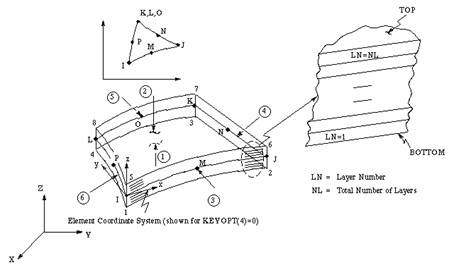Figure 1. Shell 99, 100-Layer Structural Shell 

SHELL91

Shell91 is similar Shell99 except that it allows only to 16 layers and does not allow you to input material property matrix. However, Shell91 supports plasticity, large-strain behavior and special sandwich option, whereas Shell99 does not.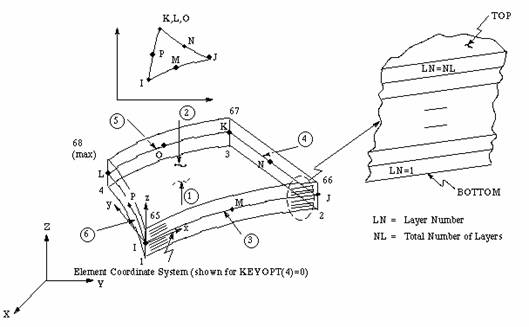Figure 2. Shell 91, 16-Layer Structural Shell 

SOLID46

Solid46 is layered version of the 8-node, 3D solid element, Solid45, with three degrees of freedom per nodes (Ux,Uy,Uz). It is designed to model layered shells or layered solids and allows up to 100 uniform- thickness layers per element. An advantage with this element type is that you can stack several elements to model more than 100 layers to allow through-to-thickness deformation slope discontinuities. The user-input constitutive matrix option is also available. Solid46 has an effective stiffness in the transverse direction permitting no-zero stresses, strains, and displacements in the transverse direction. Failure criteria may be specified. In comparison to the 8-node shells, Solid46 is a lower order element and finer meshes may be required for shell applications to provide the same accuracy as Shell91 or Shell99.

Numerical Examples

Numerical examples are presented here to highlight the accuracy of model Shell 99 and solid 46 in comparison with third-order shear deformation theory (TSDT). In the examples, the layer material constants are: E1 = 25 106 N/m; E2 = 106 N/m; G12 = 0.5 106 N/m; G23=0.2 106 N/m; n12=0.25; P0 = 1 N/m2 where subscripts 1 and 2 indicate-D directions parallel and perpendicular to the fibres, respectively.Figure 3. Solid46, 3-D Layered Structural Solid 

Numerical results and discussion

Tables 1 and 2 present the comparison of maximum displacements and stresses for laminates subjected to uniform load, respectively, for comparison with the third-order shear deformation plate theory (TSDT) of Reddy [19,20]. It can be seen that good agreement is obtained between the two models and the analytical solutions [19, 20].

The node 1 is the node to the center of the plate when the plate is modeled with by the Shell 99 element (figure 5). Node 1 and 51 are nodes to the center of the plate when the plate is modeled with Solid 46 elements (figure 4).  In addition, Fig. 6-7 shows the comparison of the deflections computed by Shell element 99 and Solid element 46, respectively. We note that results obtained with Shell 99, point of view displacement, are nearly similar those obtained with Solid 46. The percentage differences, between the two theories with respect to the continuous case are estimated according to the following formula:

% Difference= [(value of Shell99-value of Solid 46)/ value of Solide 46] × 100

Table 1. Maximum displacements for a simply supported, square (a=b) laminate with symmetrically laminated cross-ply laminate (0/90/90/0) and uniform distributed transverse load

 Element Reddy [19,20] ANSYS Ratio SHELL 99 0.0683 0.0692 1.013 SOLID 46 0.0683 0.0676 0.990

The difference between the results of Shell element 99 and Solid element 46 of center of plate, occurs as 2.37%.

Table 2. Maximum stresses for a simply supported, square (a=b) laminate with symmetrically laminated cross-ply laminate (0/90/90/0) and uniform distributed transverse load

 Stresses Solid46 (Nod 51 et 1) Shell99 (Nod 1) %Difference Top sx -8118.79 -8558.88 5.42 sy -364.39 -374.65 2.82 txy 0.000 0.240 - Botton sx 8119.00 8558.88 5.42 sy 364.16 374.65 2.82 txy 0.000 -0.240 -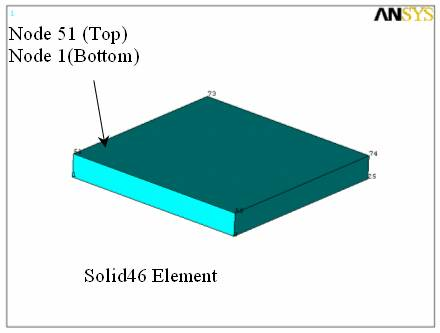Figure 4. Nodes at the center of the plate modeled with Solid 46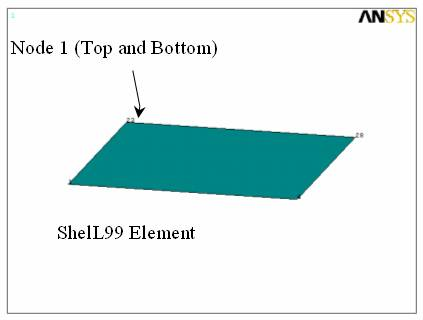Figure 5. Nodes at the center of the plate modeled with Shell 99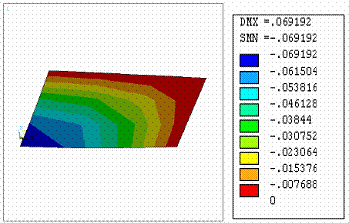Figure 6. Variation of deflection for a cross-ply laminate, through the thickness of the plate using Shell 99 Element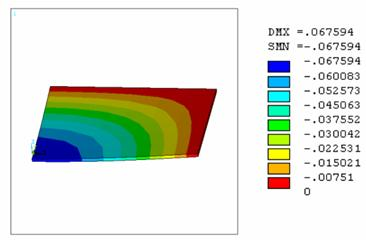Figure 7. Variation of deflection for a cross-ply laminate, through the thickness of the plate using Solid 46 Element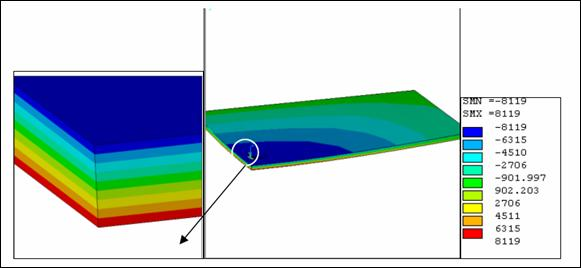Figure 8. Variation of longitudinal stress for a cross-ply laminate, through the thickness of the plate using Solid 46 Element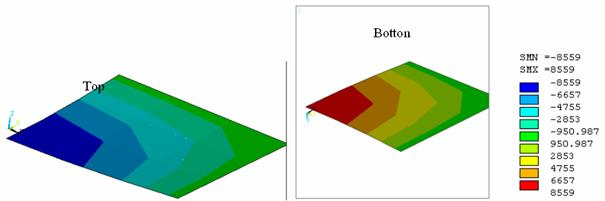Figure 9. Variation of longitudinal stress for a cross-ply laminate, through the thickness of the plate using Shell 99 Element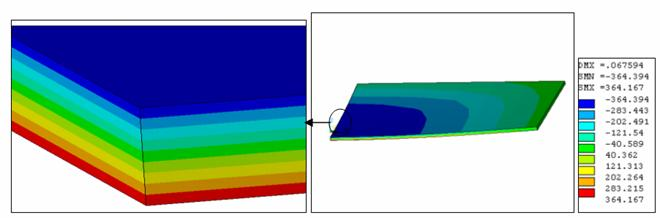Figure 10. Variation of transverse stress for a cross-ply laminate, through the thickness of the plate using Solid 46 Element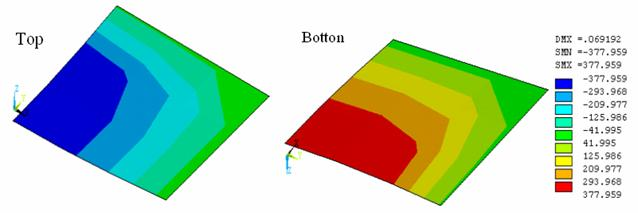Figure 11. Variation of transverse stress for a cross-ply laminate, through the thickness of the plate using Shell 99 Element

Figs. 8-11 represent the variation of the stresses in the longitudinal and transverse directions, respectively, using with the two types of elements (Shell 99 and Solid 46). The maximum tensile stress in the center of the plate is at the bottom edge (z = h/2). However, the maximum compressive stress is at the top surface (z = h/2). The percentage differences, given in the tables 2, between the two theories with respect to the continuous case are estimated according to the previous formula. From Table 2, it is seen that, in the case of center of plate, the smallest difference between the results of element 99 and Solid element 46, occurs as 2.82 %, for the stresses in the transverse directions of plate, and the highest difference is 5.42% for the stresses in the longitudinal directions of plate. Furthermore, the values of the transverse stress are lower than longitudinal stress.

Conclusions

The bending analysis of cross-ply rectangular thick plates has been performed using finite element method. For verification of the present analysis, a numerical example has been solved and compared with the third-order shear deformation plate theory and is found to be in good agreement. Based on the results reported here of cross-ply laminate plates, the following conclusions may be drawn.

1.      Deflections are nearly identical for the two types of elements: Shell 99 and Solid 46.

2.      The distribution of stress depends on the type of stacking. In addition, longitudinal stress is higher than that the transverse stress.

3.      The finite element method results are in good agreement with those obtained with the theory of Reddy [19, 20].

Finally, this method of modelling for quick solutions opens new avenues for the conception of structural composites making it possible to determine the necessary stresses instantaneously.

References

1.      Leissa A.W., Vibration of plates, NASA, SP-160, 1969.

2.      Reissner E., The effect of transverse shear deformation on the bending of elastic plates, J. Appl. Mech., 1945, 12, p. 69-77.

3.      Reissner E., On the theory of bending of elastic plates, J. Math. Phys., 1944, 23, p. 184-191.

4.      Midlin R.D., Influence of rotatory inertia and shear on flexural motions of isotropic, elastic plates, J. Appl. Mech., 1951, 18, p. 31-38.

5.      Whitney J.M., Pagano N.J., Shear deformation in heterogeneous anisotropic plates, J. Appl. Mech., 1970, 37, p. 1031-1036.

6.      Liew K.M., Xiang Y., Kitipornchai S., Transverse vibration of thick rectangular plates - I. Comprehensive sets of boundary conditions, Comp. Struct. 1993, 49, p. 1-29.

7.      Whitney J.M., Sun C.T., A higher order theory for extensional motion of laminated composites, J. Sound Vibr. 1973, 30, p. 85-97.

8.      Whitney, J.M., Sun C.T., A refined theory for laminated, anisotropic, cylindrical shells, J. Appl. Mech. 1974, 41, p. 471-6.

9.      Gautham B.P., Ganesan N., Free vibration analysis of thick spherical shells, Comp. Struct. 1992, 45(2), 307-13.

10.  Ambartsumyan S.A., Theory of anisotropic plates. 1st ed. Stamford: Technomic Publishing Co.; 1970 [Translated by T. Cheron from the first Russian Edition].

11.  Nelson R.B., Lorch D.R., A refined theory of laminated orthotropic plates, J. Appl. Mech. Trans. ASME 1974, 41, p. 177-183.

12.  Lo K.H., Christensne R.M., Wu E.M., A high order theory of plate deformation. Part I: homogeneous plates, J. Appl. Mech. 1977, 44, 663-8.

13.  Levinson M., An accurate simple theory of the statics and dynamics of elastic plates, Mech. Res. Commun. 1980, 7, 343-50.

14.  Reddy J.N., Liu C.F., A higher-order shear deformation theory of laminated elastic shells, Int. J. Eng. Sci. 1985, 23, 319-30.

15.  Lim C.W., Liew K.M., A higher order theory for vibration of shear deformable cylindrical shallow shells, Int. J. Mech. Sci. 1995, 37(3), 277-95.

16.  Liew K.M., Lim C.W., A higher-order theory for vibration analysis of constrained curvilinear shallow shells, J. Vibr. Control 1995, 1(1), 15-39.

17.  Liew K.M., Lim C.W., A higher-order theory for vibration of doubly-curved shallow shells, ASME J. Appl. Mech. 1996, 63(3), 587-93.

18.  Lim C.W., Kitipornchai S., Liew K.M., A refined higherorder theory for vibration of doubly-curved shallow shells. I Conf Mech Solids Mater Eng B 1995p. 378-83 [Singapore].

19.  Reddy J.N., Exact solutions of moderately thick laminated shells, ASCE Journal Engr. Mechanics, 1972, 110(5).

20.  Reddy J.N., Mechanics of laminated composite plate: theory and analysis, New York: CRC Press; 1997.

21.  Shimpi R.P., Patel H.G., A two variable refined plate theory for orthotropic plate analysis. Int. J. Solids Struct. 2006; 43(22-23):6783-99.

22.  Nakasone Y., Yoshimoto S., Stolarski T.A., Engineering Analysis With ANSYS Software. Elsevier Butterworth-Heinemann Linacre House, Jordan Hill, Oxford OX2 8DP  30 Corporate Drive, Burlington, MA 01803, 2006.